# New Mathematical Functions Make your Form Smarter

3202
14
09-06-2016 02:56 PM
by
New Contributor II
3 14 3,202

Survey123 for ArcGIS version 1.7 introduced support for more advanced mathematical functions, which can be called in the calculation and constraint columns of a survey question. The table below is a summary of the new mathematical functions.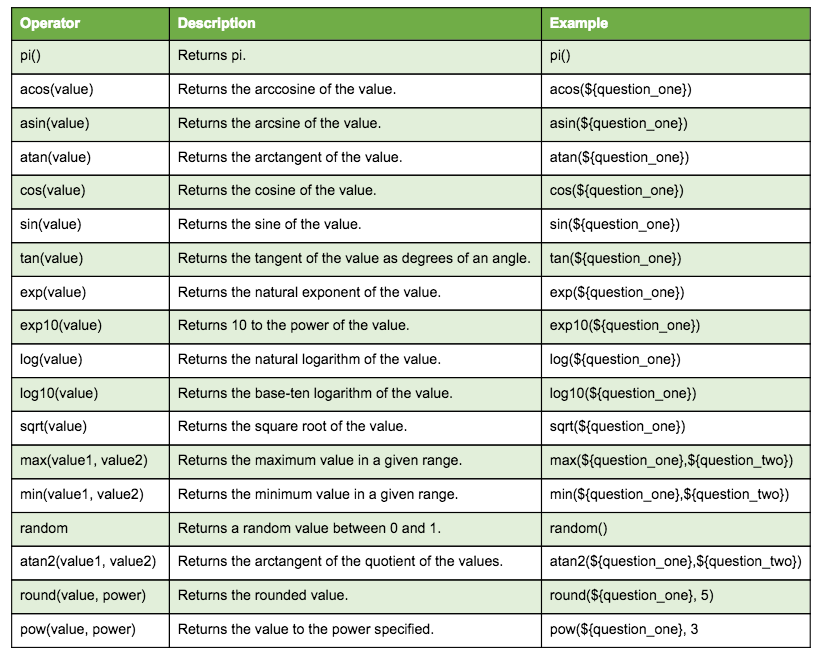With these newly supported mathematical functions, sophisticated calculations can be applied to answers in a survey. Here are just a few examples of ways that some of these functions can be incorporated into your survey.

Example 1: The area of a circular plot would be quite hard to measure directly, and without these functions you would likely need to rely on estimations or use a calculator. Now, though, the area can be calculated with this expression automatically by Survey123 for ArcGIS.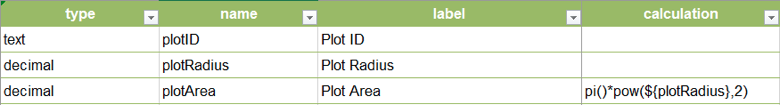Example 2: Say you are putting together a vegetation field survey. You want field users to visit a series of sites, each with 5 plots. You do not need them to sample every plot. Instead, you want them to randomly survey 2 plots in each site. The following screenshot illustrates how using the random and round functions you can make survey123 automatically present the plots to visit to the field user.

round(random()*5+0.5,0)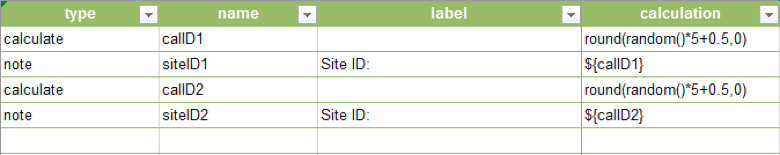Looking at the example above closely, you will notice that we use the random function first. This function picks a random number between 0 and 1, which we multiply by 5 to scale the range to five points as needed, then we add point five and round to keep the site number between 1 and 5.

Example 3: I do not intend to describe in detail the next example, but I wanted to share it with you to illustrate the level of complexity that calculations can support. This particular example was generously shared by a Survey123 user during our beta testing for 1.7 and it calculates the water flow within a pipe based on the speed of the water, diameter of the pipe and other variables.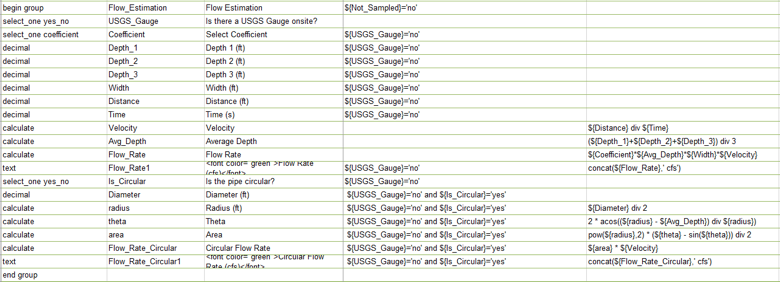If you want to learn more about how to use Mathematical functions I recommend you have a look at the following:

Try out our new mathematical functions to make your survey form smarter!

Occasional Contributor

I am attempting to use the round function and I am receiving an error message.

I would like to round to two decimal places in 'Cost per month (greater than 50" LED) (\$)' and 'Cost Annually (greater than 50" LED) (\$)'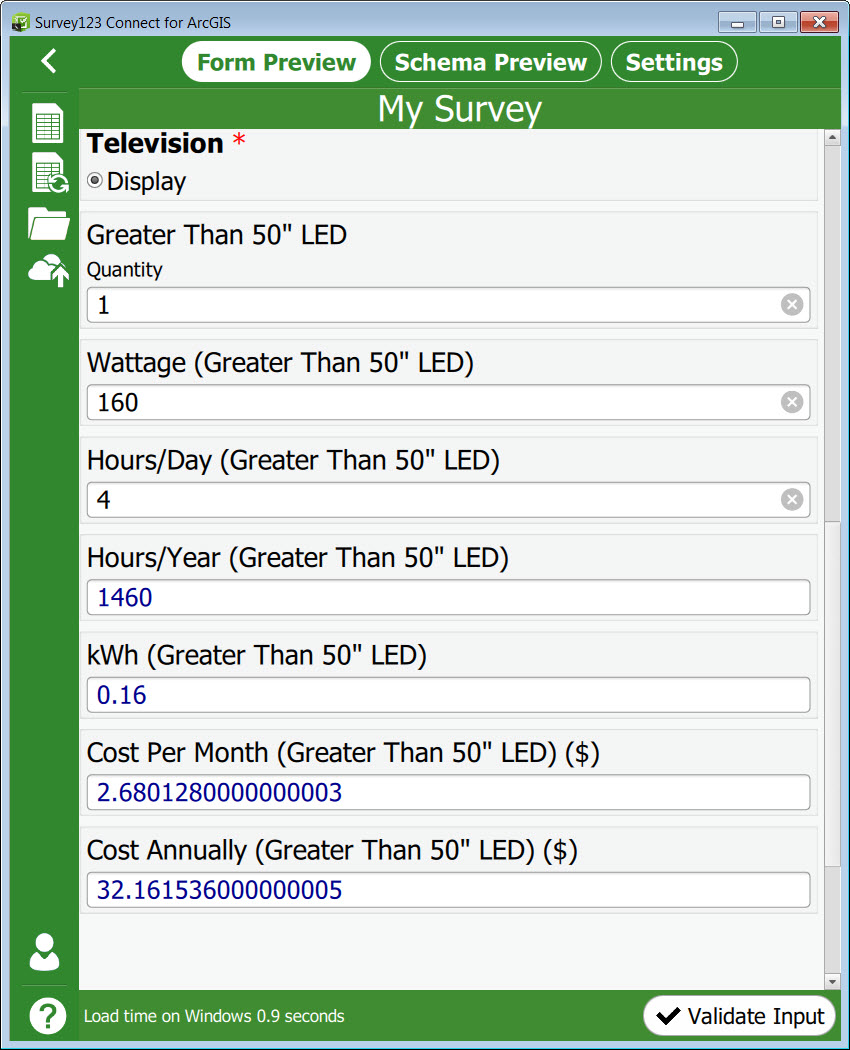Screen shot of XLS form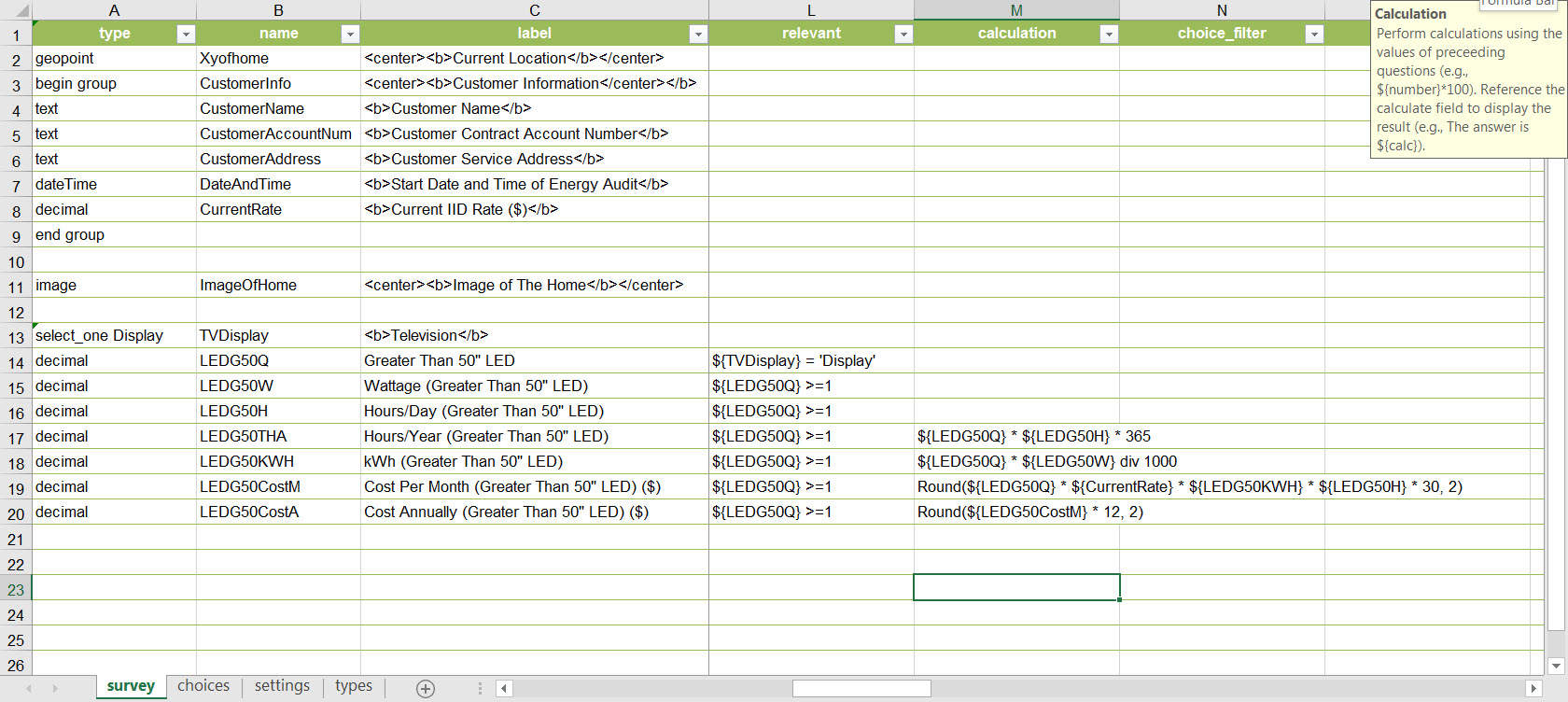Error message I am receiving: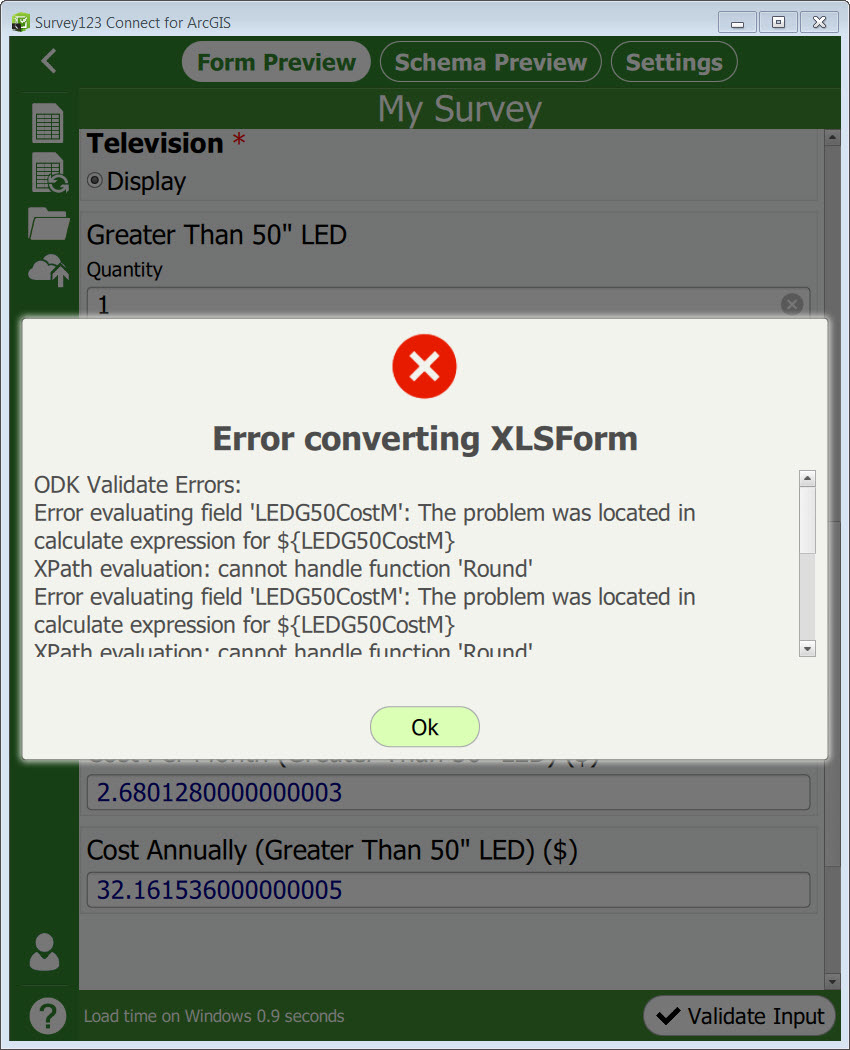by
New Contributor II

Hi Francisco:

Thank you for posting your question here.  I think you should use"round" instead of "Round" for the calculation expression.  This help document describes the expression for the round function.  I hope it helps.

Tina

Occasional Contributor

THank you Tina, you are a life saverby
New Contributor II

No Problem, I am glad to help.

New Contributor III

I am trying to write a formula that contains the sqrt() operator. However, it only seems to be returning the square root value and ignores all the other variables in the equation. For example, if my equation is 10 * sqrt(4) I would expect the answer to be 20. But in my survey it keeps coming back as 2, like it is not calculating anything else in the formula. Does anyone have an idea why this is happening?

byEsri Esteemed Contributor

Hi Shawn,

I'm not seeing the behavior you're describing. Here's the example sheet I've set up:

typenamelabelcalculation
integernum1Number:
notesq1010 * sqrt(Number)10 * sqrt(\${num1})
New Contributor III

OK, I think I know the problem. I was playing around with the Beta 2.0 Survey123 version and it seems to be a bug with that. After switching back to 1.10 it is working properly again.

byEsri Esteemed Contributor

I was using the 2.0 beta to test as well- I don't think we've had any issue appear related to the mathematical functions.

New Contributor III

Then that is very strange. I literally just opened the same form in 1.10 and it calculated correctly. I did not change anything else.

New Contributor II

Hi,

I’m trying to implement a field app in order to monitoring grapes growth in a wine farm.

In their usual approach the field workers walk in the vineyard and randomly check the number of grapes and their weight (they can’t preload a random sampling from the office).

Hence, it should be a really simple form. I created a scenario by using the Collector for ArcGis but I would like to leverage the Survey123 Connect, as well.

They have a couple of specific needs that I can’t figure out how satisfy:

1)         They would like to calculate in real time the Mean of the count of grapes and the sampling standard deviation, they need the latter in order to evaluate the size of the sampling (to decide if they have to go ahead or stop):

I can’t find the Mean and the Standard Deviation among New Mathematical Functions supported by Survey123, so I tried to “unpacked” them in several steps that I can solve with available functions. No sweat concerning the calculation of the mean. Regarding the Standard Deviation I have incorrect results… Basically I have to write a formula that for each new value that they insert, re-calculate the deviation from mean for all the values and not only for the last one. I couldn't find similar topics in the forum, do you have any suggestion for that?

2)         They would like to see in a map their real-time position while they fill the form, in order to manage sampling based on management zones. The Geopoint box in the Survey can stay active in the same page of the form?

Thanx so much for your support!

Elisabetta

this is the form preview I'm working on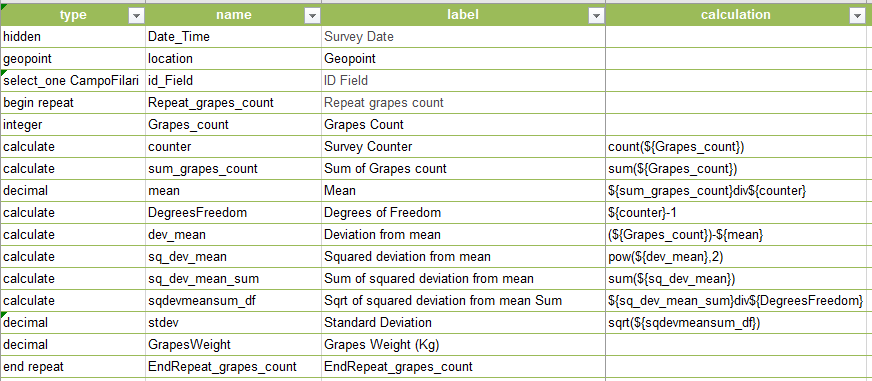byEsri Esteemed Contributor

Hi Elisabetta,

The math as you presented it is correct; the issue is that the values for the deviation from the mean and the count values for previous repeats are not updated until they are redisplayed on the form.  I've logged an issue to look into this behavior.

New Contributor II

Thank you, James

waiting for follow up on the solution!

Best,

Elisabetta

byEsri Regular Contributor

Hi James,

Is there an update on the recalculation issue discussed before? I am running into the same problem that I need to calculate Standard Deviations from samples. I tried to use the same approach as Elisabetta but facing the same problem regarding the recalculation of the mean value... It would be great if there would be a calculate button for all repeats (at once) for example or to have a calculate field that would force all fields to update the calculation. Any workaround suggestion? I am expecting to have 50 samples or more in one survey so recalculating each manually would be very awful...

Thanks!

byEsri Esteemed Contributor

Hi Nicole,

I replied to a similar discussion in